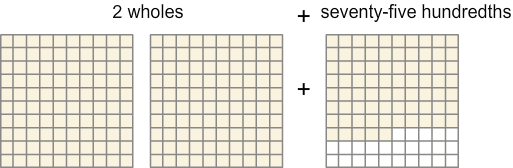# Converting Decimals to Mixed Numbers

Print Rate 0 stars
Lesson size:
Message preview:
Someone you know has shared lesson with you:

To play this lesson, click on the link below:

https://www.turtlediary.com/lesson/converting-decimals-to-mixed-numbers.html

Hope you have a good experience with this site and recommend to your friends too.

Login to rate activities and track progress.
Login to rate activities and track progress.

If you can convert a decimal to a fraction, you can easily convert a decimal with a whole part to a mixed number.

A decimal with digits to the left of the decimal point indicates there is a whole part and some parts of a whole.

For example, 2.75 or two and seventy-five hundredths means there are two whole parts and an additional seventy-five out of one hundred parts.When you are converting a number with digits both to the left and right of the decimal point, only convert the decimal part or the digits to the right of the decimal point. In other words, just leave the whole number as same and convert the decimal!

Let's take a look at some examples.

## Example 1

Write 1.2 as a fraction.

Change the decimal 0.2 to a fraction.

0.2 =
2
10

Now, reduce the fraction
2
10
to its lowest form.

2 ÷ 2
10 ÷ 2
=
1
5

So, 1.2 = 1
1
5

## Example 2

Write 3.25 as a fraction.

Change the decimal 0.25 to a fraction.

0.25 =
25
100

Now, reduce the fraction
25
100
to its lowest form.

25 ÷ 25
100 ÷ 25
=
1
4

So, 3.25 = 3
1
4

## Example 3

Write 25.4 as a fraction.

Change the decimal 0.4 to a fraction.

0.4 =
4
10

Now, reduce the fraction
4
10
to its lowest form.

4 ÷ 2
10 ÷ 2
=
2
5

So, 25.4 = 25
2
5

## Example 4

Write 130.21 as a fraction.

Change the decimal 0.21 to a fraction.

0.21 =
21
100

The fraction
21
100
is in its lowest form only, as there is no common factor of numerator and denominator.
So, 130.21 = 130
21
100

## Converting Decimals to Mixed Numbers

• A decimal with a whole part can be written as a mixed number.
• In decimals, the digits to the left of the decimal point represents the whole part and the digits to the right of the decimal point represents the decimal or fraction part.
• To convert a decimal to a mixed number, leave the whole number as same and convert the decimal part.
• Reduce fractions after changing from decimal form.

## Similar Lessons

Become premium member to get unlimited access.